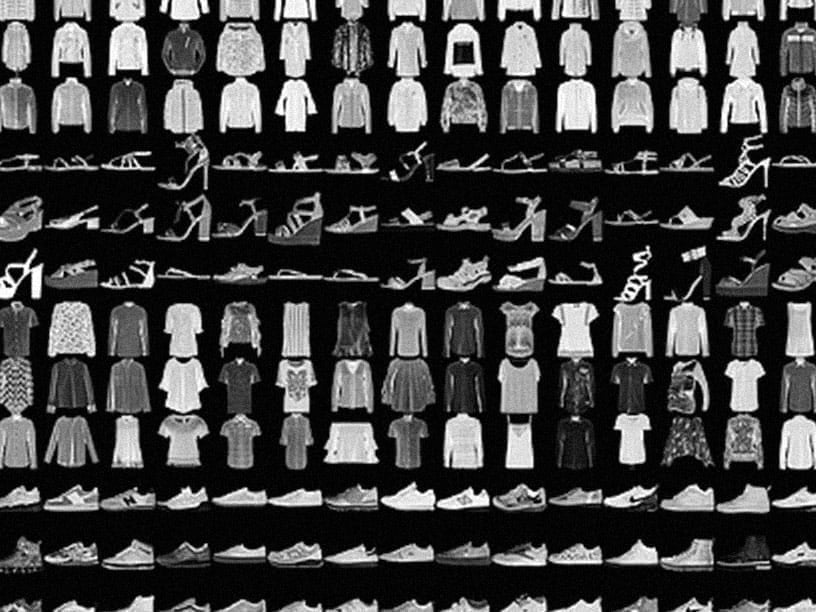# TensorFlow is an end-to-end open source platform for machine learning

TensorFlow makes it easy for beginners and experts to create machine learning models. See the sections below to get started.#### For beginners

The best place to start is with the user-friendly Sequential API. You can create models by plugging together building blocks. Run the “Hello World” example below, then visit the tutorials to learn more.

To learn ML, check out our education page. Begin with curated curriculums to improve your skills in foundational ML areas.

```import tensorflow as tf
mnist = tf.keras.datasets.mnist

x_train, x_test = x_train / 255.0, x_test / 255.0

model = tf.keras.models.Sequential([
tf.keras.layers.Flatten(input_shape=(28, 28)),
tf.keras.layers.Dense(128, activation='relu'),
tf.keras.layers.Dropout(0.2),
tf.keras.layers.Dense(10, activation='softmax')
])

loss='sparse_categorical_crossentropy',
metrics=['accuracy'])

model.fit(x_train, y_train, epochs=5)
model.evaluate(x_test, y_test)
```

#### For experts

The Subclassing API provides a define-by-run interface for advanced research. Create a class for your model, then write the forward pass imperatively. Easily author custom layers, activations, and training loops. Run the “Hello World” example below, then visit the tutorials to learn more.

```class MyModel(tf.keras.Model):
def __init__(self):
super(MyModel, self).__init__()
self.conv1 = Conv2D(32, 3, activation='relu')
self.flatten = Flatten()
self.d1 = Dense(128, activation='relu')
self.d2 = Dense(10, activation='softmax')

def call(self, x):
x = self.conv1(x)
x = self.flatten(x)
x = self.d1(x)
return self.d2(x)
model = MyModel()

logits = model(images)
loss_value = loss(logits, labels)
```

## Solutions to common problemsFor beginners

Train a neural network to classify images of clothing, like sneakers and shirts, in this fast-paced overview of a complete TensorFlow program.For experts

Train a generative adversarial network to generate images of handwritten digits, using the Keras Subclassing API.For experts
Neural machine translation with attention

Train a sequence-to-sequence model for Spanish to English translation using the Keras Subclassing API.

## News & announcements

Check out our blog for additional updates, and subscribe to our monthly TensorFlow newsletter to get the latest announcements sent directly to your inbox.September 23, 2020
Introducing TensorFlow Recommenders

We're excited to introduce TensorFlow Recommenders (TFRS), an open-source TensorFlow package that makes building, evaluating, and serving sophisticated recommender models easy.August 26, 2020
Introducing TF-Coder, a tool that writes tricky TensorFlow expressions for you!

TF-Coder is a program synthesis tool that helps you write TensorFlow code. Instead of coding tricky tensor manipulations directly, demonstrate it through an illustrative example and TF-Coder provides the corresponding code automatically. Try it yourself in a Codelab!August 11, 2020
TensorFlow Model Optimization Toolkit — Weight Clustering API

Introducing a weight clustering API, proposed and contributed by Arm. Weight clustering helps reduce the storage and transfer size of your model by replacing many unique parameter values with a smaller number of unique values.July 14, 2020
LipSync by YouTube demo with TensorFlow.js

See how well you synchronize to the lyrics of the popular hit "Dance Monkey." This in-browser experience uses the Facemesh model for estimating key points around the lips to score lip-syncing accuracy.

## Community participation

See more ways to participate in the TensorFlow community.